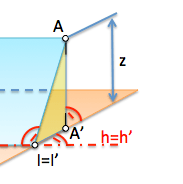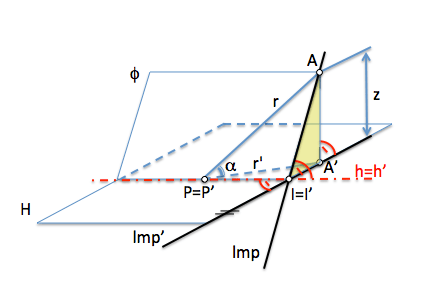# Fall lineBy studying the true magnitude of a line we saw that we could calculate in turn the angle of this line with respect to a projection plane, namely, its slope.

In a plane we can determine endless lines with different direction contained therein. If we use as a reference to mediate the horizontal plane angles such, we see that each of these lines form a different angle with the projection plane. We can ask therefore:

What is the address that will form a greater angle?

This address will determine the slope of the plane and if we get one of the infinite parallel to this direction can have a plane, We say it is their “fall line”

To see this, suppose we project the elements of a plane orthogonally on a horizontal plane. La recta de intersección del plano con el de proyección será una recta horizontal h=h’. Proyectemos un punto A del plano (proyección A’):Si determinamos una recta cualquiera del plano que pase por el punto A, y obtenemos su intersección con el plano de proyección, punto P1 de intersección con la citada recta horizontal, podremos determinar su proyección sobre el plano horizontal de proyección ya que pasará por la proyección de sus puntos (A’ y P1′).

Angle (alfa) que forma una recta con un plano es el que forma con la proyección ortogonal sobre dicho plano.vemos que podemos determinar en una figura aparte este ángulo mediante la construcción de un triángulo rectángulo (como se ve en la siguiente figura)If we obtain new lines of the plane passing through point A, they form different angles each with the horizontal projection plane. These lines have in common that the crossing point A far a certain value “z” the projection plane H.We can compare the triangles associated with these lines and allow us to obtain their corresponding angles. This figure can be seen that the angle value will be maximized when the distance is less between the projection of point A, point A ', and the intersection with the projection plane, point Pi.When this situation occurs? When the minimum distance between A’ and the horizontal line h. We need to determine the perpendicular distance therefore to this line.If we remember the theorem of three perpendicular, we see that the plane containing this minimum distance is perpendicular to the line of intersection of the plane h with the projection.Therefore we say that the straight A-I is the Straight “Max Slope” of the plane of projection plane.We see that the projection of this line in the horizontal plane is perpendicular to the horizontal plane.

Do we allow this idea to represent the plane by a single straight?

Could you determine the line of maximum slope with respect to the horizontal plane, a plane defined by three points?Sistemas_de_representacion